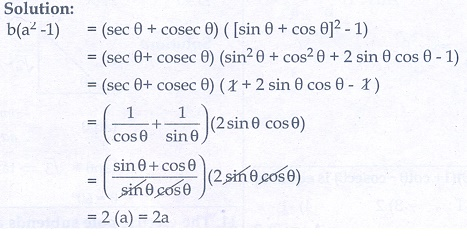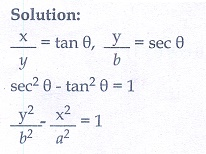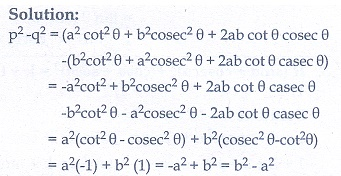Home | | Maths 10th Std | Multiple choice questions

# Multiple choice questions

Mathematics : Trigonometry: Multiple choice questions with answers / choose the correct answer with answers - Maths Book back 1 mark questions and answers with solution for Exercise Problems

Multiple choice questions

1. The value of sin2θ +is equal to

(1) tan2 θ

(2) 1

(3) cot2 θ

(4) 0

2. tanθ cosec2θ - tan θ is equal to

(1) sec θ

(2) cot2θ

(3) sin θ

(4) cotθ3. If (sin a + coseca)2 +(cos a + sec a)2  = k + tan2a + cot2, then the value of is equal to

(1) 9

(2) 7

(3) 5

(4) 34. If sin θ + cos θ = a and sec θ + cosecθ b , then the value of b(a 2 -1) is equal to

(1) 2a

(2) 3a

(3) 0

(4) 2ab5. If 5x = sec θ and 5/x = tan θthen x2 – (1/ x2) is equal to

(1) 25

(2) 1/25

(3) 5

(4) 16. If sin θ = cos θ , then 2 tan2θ + sin2θ −1 is equal to

(1) -3/2

(2) 3/2

(3) 2/3

(4) -2/37. If = a tan θ and y = b sec θ thenAns: (1)8. (1 + tan θ + sec θ)(1 + cot θ − cosecθ) is equal to

(1) 0

(2) 1

(3) 2

(4) -19. cot θ + b cosecθ = p and b cot θ +cosec θ = q then p 2 -q2  is equal to

(1) a 2 -b2

(2) b 2 -a2

(3) a 2 +b2

(4) b –a10. If the ratio of the height of a tower and the length of its shadow is √3 : 1 then the angle of elevation of the sun has measure

(1) 45°

(2) 30°

(3) 90°

(4) 60°11. The electric pole subtends an angle of 30° at a point on the same level as its foot. At a second point ‘b’ metres above the first, the depression of the foot of the tower is 60° . The height of the tower (in metres) is equal to

(1) 3b

(2) b/3

(3) b/2

(4) b/√312. A tower is 60 m height. Its shadow is x metres shorter when the sun’s altitude is 45° than when it has been 30° , then x is equal to

(1) 41.92 m

(2) 43.92 m

(3) 43 m

(4) 45.6 m13. The angle of depression of the top and bottom of 20 m tall building from the top of a multistoried building are 30° and 60° respectively. The height of the multistoried building and the distance between two buildings (in metres) is

(1) 20, 10√3

(2) 30, 5√3

(3) 20, 10

(4) 30, 10√314. Two persons are standing ‘x’ metres apart from each other and the height of the first person is double that of the other. If from the middle point of the line joining their feet an observer finds the angular elevations of their tops to be complementary, then the height of the shorter person (in metres) is

(1) √2 x

(2) / 2√2

(3) x / √2

(4) 2x15. The angle of elevation of a cloud from a point h metres above a lake is β. The angle of depression of its reflection in the lake is 45° . The height of location of the cloud from the lake is(3) h tan(45° − β)

(4) none of these

Ans: (1)Tags : Trigonometry | Mathematics , 10th Mathematics : UNIT 6 : Trigonometry
Study Material, Lecturing Notes, Assignment, Reference, Wiki description explanation, brief detail
10th Mathematics : UNIT 6 : Trigonometry : Multiple choice questions | Trigonometry | Mathematics